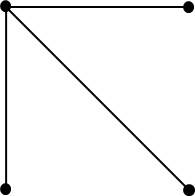## Posts Tagged ‘number-of-edges-in-a-complete-graph’

### Learning for the sheer love of it … determining the number of edges in a complete graph

September 7, 2013

As I noted in an earlier blog, even little discoveries makes one feel wonderful. Today I solved a small problem (i.e., made a little discovery) and I feel wonderful.

I am reading a wonderful book, Introduction to Graph Theory. I am at the part where it describes complete graphs. A complete graph is one where each vertex is connected to every other vertex. The author asks, How many edges are in a complete graph with N vertices? I took this as a challenge to use my reasoning skills to figure it out.

Here are three examples of complete graphs and the number of edges in each graph:Let’s arbitrarily choose one vertex. It must connect to the other (n-1) vertices. For example, in the complete graph with 4 vertices, the vertex will connect to the other 3 vertices:The remaining vertices must form a complete graph amongst themselves. Ah Ha! Now we have a simpler problem: find the number of edges in a complete graph of n-1 vertices.

I now switch on my programmer hat. I smell recursion (which I love):

```numEdges (N) {
if (N == 2) then return 1
else return (N-1) + numEdges (N-1)
```

Let’s check that program:

if N = 2, then num edges = 2
if N = 3, then num edges = 2 + 1 = 3
if N = 4, then num edges = 3 + 3 = 6
if N = 5, then num edges = 4 + 6 = 10
if N = 6, then num edges = 5 + 10 = 15
if N = 7, then num edges = 6 + 15 = 21
if N = 8, then num edges = 7 + 21 = 28

Wow! That is cool.

One problem, however. What are the number of edges when, say, N = 1000?

I could implement the above program and set it to solve N=1000. But I know the mathematicians would tell me: Find a formula.

Okay, let’s see … to find the number of edges in a complete graph with N vertices, I must add these numbers:

(N-1) + (N-2) + (N-3) + … + 1

or equivalently:

1 + 2 + … + (N-1)

I remember from school days a formula for the sum of the first N integers:

n(n+1)/2

Of course, I want the sum of the first N-1 integers, so I substitute “n” with N-1:

(N-1)((N-1)+1)/2
= (N-1)(N-1+1)/2
= (N-1)(N)/2
= N(N-1)/2

Let’s check:

if N = 2, then 2(1)/2 = 1
if N = 3, then num edges = 3(2)/2 = 6
if N = 4, then num edges = 4(3)/2 = 6
if N = 5, then num edges = 5(4)/2 = 10
if N = 6, then num edges = 6(5)/2 = 15
if N = 7, then num edges = 7(6)/2 = 21
if N = 8, then num edges = 8(7)/2 = 28

Yea!

I am pretty proud of myself. I solved it, not because it was a homework problem or a work problem. I solved it purely for the love of learning. I figured it out by simple reasoning.

I feel wonderful.